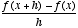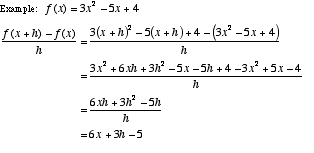index: click on a letter A B C D E F G H I J K L M N O P Q R S T U V W X Y Z A to Z index index: subject areas numbers & symbols sets, logic, proofs geometry algebra trigonometry advanced algebra & pre-calculus calculus advanced topics probability & statistics real world applications multimedia entrieswww.mathwords.com about mathwords website feedback

 Difference Quotient For a function f, the formula. This formula computes the slope of the secant line through two points on the graph of f. These are the points with x-coordinates x and x + h. The difference quotient is used in the definition the derivative.